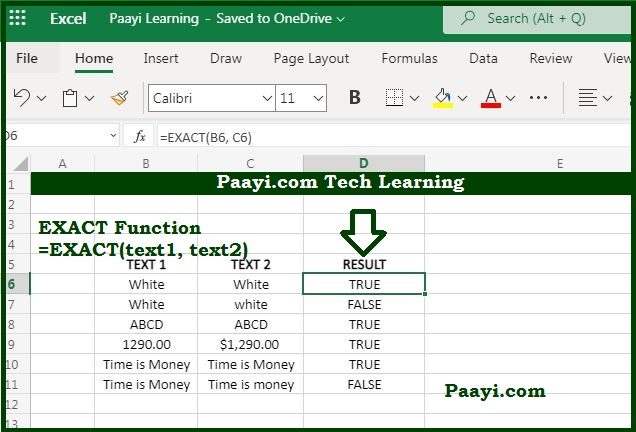# Learn How to Use Microsoft Excel EXACT Function

Written by | 0 Comments | 507 Views

In this article, you will learn how to use the Microsoft Excel EXACT function and its prime function in Microsoft Excel. You will also get to know the Microsoft Excel EXACT function return value and syntax with the help of some examples.

## Microsoft Excel EXACT Function

The primary purpose of the Microsoft Excel EXACT function is to compare two text things. So with the help of the EXACT function, you can compare the two text strings, taking into account upper and lower case, and it will return the TRUE if they are identical and FALSE if it does not match. It should be noted here that, EXACT function is case-sensitive.

### EXACT Function Return Value

It will return a boolean value - TRUE or FALSE.

### EXACT Function Syntax

= EXACT (text1, text2)

Where the arguments:

• text1: It is the first text string you want to compare.
• text2: It is the second text string you want to compare.

## How to Use Microsoft Excel EXACT Function?As we know, the primary purpose of the MS Excel EXACT function is to compare two text things in a case-sensitive manner. In case the two text-strings are precisely the same, it will return the TRUE, and if not, it will return the FALSE.

Let us understand the EXACT function, with the help of examples given below:

• = EXACT ("banana", "banana") - This will return the TRUE, as the case is exactly matched.
• = EXACT("BANANA", "banana") - This will return FALSE, as case is not matched.